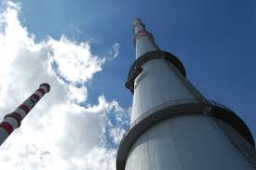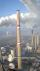# Powerplant chimney

From the building window at the height of 7.5 m, we can see the top of the factory chimney at an altitude angle of 76° 30 ′. We can see the chimney base from the same place at a depth angle of 5° 50 ′. How tall is the chimney?

x =  313.2801 m

### Step-by-step explanation:Did you find an error or inaccuracy? Feel free to write us. Thank you!Tips to related online calculators
Check out our ratio calculator.

#### You need to know the following knowledge to solve this word math problem:

We encourage you to watch this tutorial video on this math problem:

## Related math problems and questions:

• Depth anglesAt the top of the mountain stands a castle, which has a tower 30 meters high. We see the crossroad in the valley from the top of the tower and heel at depth angles of 32° 50 'and 30° 10'. How high is the top of the mountain above the crossroad
• Balloon and bridgeFrom the balloon, which is 92 m above the bridge, one end of the bridge is seen at a depth angle of 37° and the second end at depth angle 30° 30 '. Calculate the length of the bridge.
• TreeBetween points A and B is 50m. From A we see a tree at an angle 18°. From point B we see the tree in three times bigger angle. How tall is a tree?
• Angles of elevationFrom points A and B on level ground, the angles of elevation of the top of a building are 25° and 37° respectively. If |AB| = 57m, calculate, to the nearest meter, the distances of the top of the building from A and B if they are both on the same side of
• Tower's viewFrom the church tower's view at the height of 65 m, the top of the house can be seen at a depth angle of alpha = 45° and its bottom at a depth angle of beta = 58°. Calculate the height of the house and its distance from the church.
• TV towerCalculate the height of the television tower if an observer standing 430 m from the base of the tower sees the peak at an altitude angle of 23°?
• BuildingThe building I focused at an angle 30°. When I moved 5 m building I focused at an angle 45°. What is the height of the building?
• An observerAn observer standing west of the tower sees its top at an altitude angle of 45 degrees. After moving 50 meters to the south, he sees its top at an altitude angle of 30 degrees. How tall is the tower?
• Two boatsTwo boats are located from a height of 150m above the surface of the lake at depth angles of 57° and 39°. Find the distance of both boats if the sighting device and both ships are in a plane perpendicular to the surface of the lake.
• TrianglesFind out whether given sizes of the angles can be interior angles of a triangle: a) 23°10',84°30',72°20' b) 90°,41°33',48°37' c) 14°51',90°,75°49' d) 58°58',59°59',60°3'
• ChimneyThe lower circumference of the chimney is 12.57 m, and the top circumference is 5.655 m. The slope of the walls is 87°. Find the height of the chimney.The ladder touch on a wall at a height of 7.5 m. The angle of the inclination of the ladder is 76°. How far is the lower end of the ladder from the wall?How high is the building that throws horizontal shadow 85.6 m long at angle 34°12'?Calculate the area of triangle ABC, if given by alpha = 49°, beta = 31°, and the height on the c side is 9cm.The observer sees the tower's base 96 meters high at a depth of 30 degrees and 10 minutes and the top of the tower at a depth of 20 degrees and 50 minutes. How high is the observer above the horizontal plane on which the tower stands?A military fighter flies at an altitude of 10 km. The ground position was aimed at an altitude angle of 23° and 12 seconds later at an altitude angle of 27°. Calculate the speed of the fighter in km/h.From the observation tower at a height of 105 m above sea level, the ship is aimed at a depth angle of 1° 49´. How far is the ship from the base of the tower?